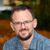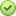# Export multiple tables in GDB to Excel with a new sheet for each table

4289
11
05-16-2018 09:05 AMOccasional Contributor II

Researching to see if there is a way to use Python to export multiple tables in a File Geodatabase to a single Excel file. I would like for each sheet name to have the same name as the table.

My current situation is that I ran a Python script to take all the domains a GDB and create a table within the GDB.

The article referenced from:

``````def main():
import arcpy, os
arcpy.env.overwriteOutput = True

for dom in arcpy.da.ListDomains(gdb):
if dom.domainType == 'CodedValue':
arcpy.DomainToTable_management(in_workspace=gdb,
domain_name=dom.name,
out_table=os.path.join(gdb, dom.name),
code_field="item",
description_field="descrip",
configuration_keyword="")

print " - domain '{0}' of type '{1}' exported to table".format(dom.name, dom.domainType)
else:
print " - domain '{0}' of type '{1}' skipped".format(dom.name, dom.domainType)

if __name__ == '__main__':
main()‍‍‍‍‍‍‍‍‍‍‍‍‍‍‍‍‍‍‍‍``````
Brian
Tags (2)
11 RepliesbyMVP Esteemed Contributor

I'm in the same boat: I have multiple tables in a fgdb and would like to export them to a workbook as individual worksheets. Just moments ago, I installed the xlsxwriter module as a package in ArcGIS Pro.  That's the easy part.  Now I'm working on figuring it out.  See: Getting Started with XlsxWriter — XlsxWriter Documentation   ( I just create my Hello.xls...)

Hey Dan, Joshua or Curtis- ever work with xlsxwriter?

That should just about do it....byMVP Esteemed Contributor

yes ... but not tonightbyMVP Esteemed ContributorThat should just about do it....byMVP Esteemed ContributorbyMVP Esteemed Contributor

Thanks.  I'll take a look in the morning.

That should just about do it....byMVP Esteemed Contributor

Getting pretty close, but not quite there.  For some reason, I can only get pandas to write one of my tables out to the target excel spreadhheet, and that depends on where I put writer.save() function:

``````import pandas as pd
import arcpy
from arcpy import env
fields = ['TaxingEntity', 'ProjectID']
targetXL = r'J:\RDA\junk.xls'
#
arcpy.env.workspace = r'J:\RDA\Temp2.gdb'
#tables = arcpy.ListTables()
tables = ['Project101','Project102', 'Project103' ]
for table in tables:
if table == 'AllTables_5_16':
pass
elif table == 'NeedTaxEntRecords':
pass
elif table == 'BlakesTaxinEntities':
pass
else:
inTable = table
print (table)
tableArray = arcpy.da.TableToNumPyArray(inTable,fields)
print (tableArray)
writer = pd.ExcelWriter(targetXL)
df = pd.DataFrame(data=tableArray)
df.to_excel(targetXL,table)
writer.save()
del df‍‍‍‍‍‍‍‍‍‍‍‍‍‍‍‍‍‍‍‍‍‍‍‍‍‍``````

In this example of my code, I'm only working with 3 of the 100+ tables which is why line 8 is commented and line 9 creates a list of table names.  The problem child seems to be line 25 writer.save(): at it's current location inside the for loop, I only get Project101 written to the target, and I get an error:

IndexError: list index out of range

If I comment it out or put it outside the for loop, I don't get any errors, but I only get Project 103 to get written to the target.

Perhaps a second (or third) set of eyes might see what the issue could be?

That should just about do it....byMVP Esteemed Contributor

My guess is this line is messing it up:

``df.to_excel(targetXL,table)‍``

Instead of passing the XLS file path, try passing the writer itself.byMVP Esteemed Contributor

Following pandas.DataFrame.to_excel — pandas 0.23.0 documentation , it shows:

``````>>> writer = pd.ExcelWriter('output.xlsx')
>>> df1.to_excel(writer,'Sheet1')
>>> df2.to_excel(writer,'Sheet2')
>>> writer.save()‍‍‍‍``````

However, when I use 'writer' as shown in lines 1 & 2 (line 24 in my script) the target excel work book does get created at all. However, when I use targetXL , it does get created,with only one sheet.  Weird....

When I take the process out of the loop, and only work with 1 table it works as you and the documentation suggests:

``````import pandas as pd
import arcpy
from arcpy import env
fields = ['TaxingEntity', 'ProjectID']
targetXL = r'J:\RDA\junk.xls'
#
arcpy.env.workspace = r'J:\RDA\Temp2.gdb'

table = 'Project101'    # just one, not a list now....

inTable = table
tableArray = arcpy.da.TableToNumPyArray(inTable,fields)
writer = pd.ExcelWriter(targetXL)
df = pd.DataFrame(tableArray)
df.to_excel(writer,table)
writer.save()‍‍‍‍‍‍‍‍‍‍‍‍‍‍‍‍``````

When I put print() functions in along the way, I can see each array, and each subsequent dataframe while in the original loop; again weird.  I'll keep hacking.....

That should just about do it....byMVP Esteemed Contributor

Ha.... Got it....  Notice below where I place the writer variable: outside the for loop.  What was happening is the target xls would get re-initialized each time through the loop.  Now that I set it up outside the loop, it runs like a champ!  Fun stuff!  And I'm getting paid for it!

``````import pandas as pd
import arcpy
from arcpy import env
fields = ['TaxingEntity', 'ProjectID']
targetXL = r'J:\RDA\ToBlake.xls' ##### <- set up the target outside the loop
#
arcpy.env.workspace = r'J:\RDA\Temp2.gdb'
tables = arcpy.ListTables()
writer = pd.ExcelWriter(targetXL)
for table in tables:
if table == 'AllTables_5_16':
pass
elif table == 'NeedTaxEntRecords':
pass
elif table == 'BlakesTaxinEntities':
pass
else:
inTable = table
tableArray = arcpy.da.TableToNumPyArray(inTable,fields)
df = pd.DataFrame(tableArray)
df.to_excel(writer,table)
del tableArray
del df
writer.save()‍‍‍‍‍‍‍‍‍‍‍‍‍‍‍‍‍‍‍‍‍‍‍‍``````